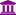# "FP Growth - is there a

Member, University Professor Posts: 6University Professor
edited May 2019 in Help
I am running FP-Growth through Create Association Rules.
There is one item that occurs very frequently because it is simply very popular, and so produces a lot of spurious or "uninteresting" rules. Is there any way to specify an item should not be used, rather than inisitng that an item must be used?
Tagged:

• Member, University Professor Posts: 6University Professor
edited March 2019 Solution Accepted
Update: I've realised that I can get the same effect by inserting one or more Replace operators between the example set and FP-Growth, and replacing the unwanted terms with nothing.
But any more elegant solutions, or advice on more complex subsetting, still appreciated.

• Administrator, Moderator, Employee, RapidMiner Certified Analyst, RapidMiner Certified Expert, Community Manager, RMResearcher, Member, University Professor Posts: 1,751RM Founder
`<?xml version="1.0" encoding="UTF-8"?><process version="9.2.000"><br>&nbsp; <context><br>&nbsp;&nbsp;&nbsp; <input/><br>&nbsp;&nbsp;&nbsp; <output/><br>&nbsp;&nbsp;&nbsp; <macros/><br>&nbsp; </context><br>&nbsp; <operator activated="true" class="process" compatibility="9.2.000" expanded="true" name="Process"><br>&nbsp;&nbsp;&nbsp; <parameter key="logverbosity" value="init"/><br>&nbsp;&nbsp;&nbsp; <parameter key="random_seed" value="2001"/><br>&nbsp;&nbsp;&nbsp; <parameter key="send_mail" value="never"/><br>&nbsp;&nbsp;&nbsp; <parameter key="notification_email" value=""/><br>&nbsp;&nbsp;&nbsp; <parameter key="process_duration_for_mail" value="30"/><br>&nbsp;&nbsp;&nbsp; <parameter key="encoding" value="UTF-8"/><br>&nbsp;&nbsp;&nbsp; <process expanded="true"><br>&nbsp;&nbsp;&nbsp;&nbsp;&nbsp; <operator activated="true" class="retrieve" compatibility="9.2.000" expanded="true" height="68" name="Retrieve Golf" width="90" x="45" y="34"><br>&nbsp;&nbsp;&nbsp;&nbsp;&nbsp;&nbsp;&nbsp; <parameter key="repository_entry" value="//Samples/data/Golf"/><br>&nbsp;&nbsp;&nbsp;&nbsp;&nbsp; </operator><br>&nbsp;&nbsp;&nbsp;&nbsp;&nbsp; <operator activated="true" class="discretize_by_frequency" compatibility="9.2.000" expanded="true" height="103" name="Discretize" width="90" x="179" y="34"><br>&nbsp;&nbsp;&nbsp;&nbsp;&nbsp;&nbsp;&nbsp; <parameter key="return_preprocessing_model" value="false"/><br>&nbsp;&nbsp;&nbsp;&nbsp;&nbsp;&nbsp;&nbsp; <parameter key="create_view" value="false"/><br>&nbsp;&nbsp;&nbsp;&nbsp;&nbsp;&nbsp;&nbsp; <parameter key="attribute_filter_type" value="value_type"/><br>&nbsp;&nbsp;&nbsp;&nbsp;&nbsp;&nbsp;&nbsp; <parameter key="attribute" value=""/><br>&nbsp;&nbsp;&nbsp;&nbsp;&nbsp;&nbsp;&nbsp; <parameter key="attributes" value=""/><br>&nbsp;&nbsp;&nbsp;&nbsp;&nbsp;&nbsp;&nbsp; <parameter key="use_except_expression" value="false"/><br>&nbsp;&nbsp;&nbsp;&nbsp;&nbsp;&nbsp;&nbsp; <parameter key="value_type" value="numeric"/><br>&nbsp;&nbsp;&nbsp;&nbsp;&nbsp;&nbsp;&nbsp; <parameter key="use_value_type_exception" value="false"/><br>&nbsp;&nbsp;&nbsp;&nbsp;&nbsp;&nbsp;&nbsp; <parameter key="except_value_type" value="real"/><br>&nbsp;&nbsp;&nbsp;&nbsp;&nbsp;&nbsp;&nbsp; <parameter key="block_type" value="value_series"/><br>&nbsp;&nbsp;&nbsp;&nbsp;&nbsp;&nbsp;&nbsp; <parameter key="use_block_type_exception" value="false"/><br>&nbsp;&nbsp;&nbsp;&nbsp;&nbsp;&nbsp;&nbsp; <parameter key="except_block_type" value="value_series_end"/><br>&nbsp;&nbsp;&nbsp;&nbsp;&nbsp;&nbsp;&nbsp; <parameter key="invert_selection" value="false"/><br>&nbsp;&nbsp;&nbsp;&nbsp;&nbsp;&nbsp;&nbsp; <parameter key="include_special_attributes" value="false"/><br>&nbsp;&nbsp;&nbsp;&nbsp;&nbsp;&nbsp;&nbsp; <parameter key="use_sqrt_of_examples" value="false"/><br>&nbsp;&nbsp;&nbsp;&nbsp;&nbsp;&nbsp;&nbsp; <parameter key="number_of_bins" value="3"/><br>&nbsp;&nbsp;&nbsp;&nbsp;&nbsp;&nbsp;&nbsp; <parameter key="range_name_type" value="long"/><br>&nbsp;&nbsp;&nbsp;&nbsp;&nbsp;&nbsp;&nbsp; <parameter key="automatic_number_of_digits" value="true"/><br>&nbsp;&nbsp;&nbsp;&nbsp;&nbsp;&nbsp;&nbsp; <parameter key="number_of_digits" value="-1"/><br>&nbsp;&nbsp;&nbsp;&nbsp;&nbsp; </operator><br>&nbsp;&nbsp;&nbsp;&nbsp;&nbsp; <operator activated="true" class="nominal_to_binominal" compatibility="9.2.000" expanded="true" height="103" name="Nominal to Binominal" width="90" x="313" y="34"><br>&nbsp;&nbsp;&nbsp;&nbsp;&nbsp;&nbsp;&nbsp; <parameter key="return_preprocessing_model" value="false"/><br>&nbsp;&nbsp;&nbsp;&nbsp;&nbsp;&nbsp;&nbsp; <parameter key="create_view" value="false"/><br>&nbsp;&nbsp;&nbsp;&nbsp;&nbsp;&nbsp;&nbsp; <parameter key="attribute_filter_type" value="all"/><br>&nbsp;&nbsp;&nbsp;&nbsp;&nbsp;&nbsp;&nbsp; <parameter key="attribute" value=""/><br>&nbsp;&nbsp;&nbsp;&nbsp;&nbsp;&nbsp;&nbsp; <parameter key="attributes" value=""/><br>&nbsp;&nbsp;&nbsp;&nbsp;&nbsp;&nbsp;&nbsp; <parameter key="use_except_expression" value="false"/><br>&nbsp;&nbsp;&nbsp;&nbsp;&nbsp;&nbsp;&nbsp; <parameter key="value_type" value="nominal"/><br>&nbsp;&nbsp;&nbsp;&nbsp;&nbsp;&nbsp;&nbsp; <parameter key="use_value_type_exception" value="false"/><br>&nbsp;&nbsp;&nbsp;&nbsp;&nbsp;&nbsp;&nbsp; <parameter key="except_value_type" value="file_path"/><br>&nbsp;&nbsp;&nbsp;&nbsp;&nbsp;&nbsp;&nbsp; <parameter key="block_type" value="single_value"/><br>&nbsp;&nbsp;&nbsp;&nbsp;&nbsp;&nbsp;&nbsp; <parameter key="use_block_type_exception" value="false"/><br>&nbsp;&nbsp;&nbsp;&nbsp;&nbsp;&nbsp;&nbsp; <parameter key="except_block_type" value="single_value"/><br>&nbsp;&nbsp;&nbsp;&nbsp;&nbsp;&nbsp;&nbsp; <parameter key="invert_selection" value="false"/><br>&nbsp;&nbsp;&nbsp;&nbsp;&nbsp;&nbsp;&nbsp; <parameter key="include_special_attributes" value="false"/><br>&nbsp;&nbsp;&nbsp;&nbsp;&nbsp;&nbsp;&nbsp; <parameter key="transform_binominal" value="false"/><br>&nbsp;&nbsp;&nbsp;&nbsp;&nbsp;&nbsp;&nbsp; <parameter key="use_underscore_in_name" value="false"/><br>&nbsp;&nbsp;&nbsp;&nbsp;&nbsp; </operator><br>&nbsp;&nbsp;&nbsp;&nbsp;&nbsp; <operator activated="true" class="concurrency:fp_growth" compatibility="9.2.000" expanded="true" height="82" name="FP-Growth" width="90" x="447" y="34"><br>&nbsp;&nbsp;&nbsp;&nbsp;&nbsp;&nbsp;&nbsp; <parameter key="input_format" value="items in dummy coded columns"/><br>&nbsp;&nbsp;&nbsp;&nbsp;&nbsp;&nbsp;&nbsp; <parameter key="item_separators" value="|"/><br>&nbsp;&nbsp;&nbsp;&nbsp;&nbsp;&nbsp;&nbsp; <parameter key="use_quotes" value="false"/><br>&nbsp;&nbsp;&nbsp;&nbsp;&nbsp;&nbsp;&nbsp; <parameter key="quotes_character" value="&quot;"/><br>&nbsp;&nbsp;&nbsp;&nbsp;&nbsp;&nbsp;&nbsp; <parameter key="escape_character" value="\"/><br>&nbsp;&nbsp;&nbsp;&nbsp;&nbsp;&nbsp;&nbsp; <parameter key="trim_item_names" value="true"/><br>&nbsp;&nbsp;&nbsp;&nbsp;&nbsp;&nbsp;&nbsp; <parameter key="min_requirement" value="support"/><br>&nbsp;&nbsp;&nbsp;&nbsp;&nbsp;&nbsp;&nbsp; <parameter key="min_support" value="0.95"/><br>&nbsp;&nbsp;&nbsp;&nbsp;&nbsp;&nbsp;&nbsp; <parameter key="min_frequency" value="100"/><br>&nbsp;&nbsp;&nbsp;&nbsp;&nbsp;&nbsp;&nbsp; <parameter key="min_items_per_itemset" value="1"/><br>&nbsp;&nbsp;&nbsp;&nbsp;&nbsp;&nbsp;&nbsp; <parameter key="max_items_per_itemset" value="0"/><br>&nbsp;&nbsp;&nbsp;&nbsp;&nbsp;&nbsp;&nbsp; <parameter key="max_number_of_itemsets" value="1000000"/><br>&nbsp;&nbsp;&nbsp;&nbsp;&nbsp;&nbsp;&nbsp; <parameter key="find_min_number_of_itemsets" value="true"/><br>&nbsp;&nbsp;&nbsp;&nbsp;&nbsp;&nbsp;&nbsp; <parameter key="min_number_of_itemsets" value="100"/><br>&nbsp;&nbsp;&nbsp;&nbsp;&nbsp;&nbsp;&nbsp; <parameter key="max_number_of_retries" value="15"/><br>&nbsp;&nbsp;&nbsp;&nbsp;&nbsp;&nbsp;&nbsp; <parameter key="requirement_decrease_factor" value="0.9"/><br>&nbsp;&nbsp;&nbsp;&nbsp;&nbsp;&nbsp;&nbsp; <enumeration key="must_contain_list"/><br>&nbsp;&nbsp;&nbsp;&nbsp;&nbsp; </operator><br>&nbsp;&nbsp;&nbsp;&nbsp;&nbsp; <operator activated="true" class="create_association_rules" compatibility="9.2.000" expanded="true" height="82" name="Create Association Rules" width="90" x="581" y="34"><br>&nbsp;&nbsp;&nbsp;&nbsp;&nbsp;&nbsp;&nbsp; <parameter key="criterion" value="confidence"/><br>&nbsp;&nbsp;&nbsp;&nbsp;&nbsp;&nbsp;&nbsp; <parameter key="min_confidence" value="0.5"/><br>&nbsp;&nbsp;&nbsp;&nbsp;&nbsp;&nbsp;&nbsp; <parameter key="min_criterion_value" value="0.8"/><br>&nbsp;&nbsp;&nbsp;&nbsp;&nbsp;&nbsp;&nbsp; <parameter key="gain_theta" value="2.0"/><br>&nbsp;&nbsp;&nbsp;&nbsp;&nbsp;&nbsp;&nbsp; <parameter key="laplace_k" value="1.0"/><br>&nbsp;&nbsp;&nbsp;&nbsp;&nbsp; </operator><br>&nbsp;&nbsp;&nbsp;&nbsp;&nbsp; <operator activated="true" class="converters:rules_2_example_set" compatibility="0.5.000" expanded="true" height="82" name="Association Rules to ExampleSet" width="90" x="715" y="34"/><br>&nbsp;&nbsp;&nbsp;&nbsp;&nbsp; <operator activated="true" class="filter_examples" compatibility="9.2.000" expanded="true" height="103" name="Filter Examples" width="90" x="849" y="34"><br>&nbsp;&nbsp;&nbsp;&nbsp;&nbsp;&nbsp;&nbsp; <parameter key="parameter_expression" value=""/><br>&nbsp;&nbsp;&nbsp;&nbsp;&nbsp;&nbsp;&nbsp; <parameter key="condition_class" value="custom_filters"/><br>&nbsp;&nbsp;&nbsp;&nbsp;&nbsp;&nbsp;&nbsp; <parameter key="invert_filter" value="false"/><br>&nbsp;&nbsp;&nbsp;&nbsp;&nbsp;&nbsp;&nbsp; <list key="filters_list"><br>&nbsp;&nbsp;&nbsp;&nbsp;&nbsp;&nbsp;&nbsp;&nbsp;&nbsp; <parameter key="filters_entry_key" value="Premises.does_not_contain.Outlook"/><br>&nbsp;&nbsp;&nbsp;&nbsp;&nbsp;&nbsp;&nbsp;&nbsp;&nbsp; <parameter key="filters_entry_key" value="Conclusion.does_not_contain.Outlook"/><br>&nbsp;&nbsp;&nbsp;&nbsp;&nbsp;&nbsp;&nbsp; </list><br>&nbsp;&nbsp;&nbsp;&nbsp;&nbsp;&nbsp;&nbsp; <parameter key="filters_logic_and" value="true"/><br>&nbsp;&nbsp;&nbsp;&nbsp;&nbsp;&nbsp;&nbsp; <parameter key="filters_check_metadata" value="true"/><br>&nbsp;&nbsp;&nbsp;&nbsp;&nbsp; </operator><br>&nbsp;&nbsp;&nbsp;&nbsp;&nbsp; <connect from_op="Retrieve Golf" from_port="output" to_op="Discretize" to_port="example set input"/><br>&nbsp;&nbsp;&nbsp;&nbsp;&nbsp; <connect from_op="Discretize" from_port="example set output" to_op="Nominal to Binominal" to_port="example set input"/><br>&nbsp;&nbsp;&nbsp;&nbsp;&nbsp; <connect from_op="Nominal to Binominal" from_port="example set output" to_op="FP-Growth" to_port="example set"/><br>&nbsp;&nbsp;&nbsp;&nbsp;&nbsp; <connect from_op="FP-Growth" from_port="frequent sets" to_op="Create Association Rules" to_port="item sets"/><br>&nbsp;&nbsp;&nbsp;&nbsp;&nbsp; <connect from_op="Create Association Rules" from_port="rules" to_op="Association Rules to ExampleSet" to_port="rules input"/><br>&nbsp;&nbsp;&nbsp;&nbsp;&nbsp; <connect from_op="Association Rules to ExampleSet" from_port="example set" to_op="Filter Examples" to_port="example set input"/><br>&nbsp;&nbsp;&nbsp;&nbsp;&nbsp; <connect from_op="Filter Examples" from_port="example set output" to_port="result 1"/><br>&nbsp;&nbsp;&nbsp;&nbsp;&nbsp; <portSpacing port="source_input 1" spacing="0"/><br>&nbsp;&nbsp;&nbsp;&nbsp;&nbsp; <portSpacing port="sink_result 1" spacing="0"/><br>&nbsp;&nbsp;&nbsp;&nbsp;&nbsp; <portSpacing port="sink_result 2" spacing="42"/><br>&nbsp;&nbsp;&nbsp; </process><br>&nbsp; </operator><br></process>`